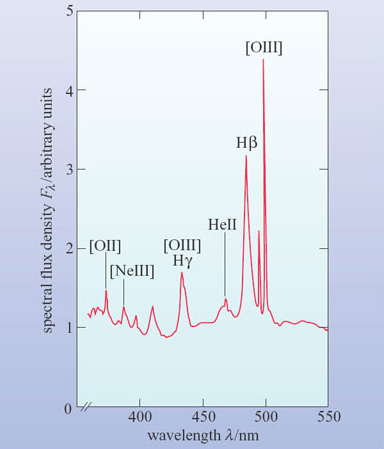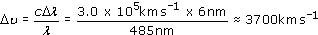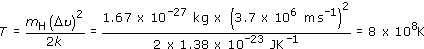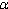Science, Maths & Technology

### Become an OU studentIntroduction to active galaxies

Start this free course now. Just create an account and sign in. Enrol and complete the course for a free statement of participation or digital badge if available.

# Active galaxies

Figure 6 shows a schematic optical spectrum of an active galaxy. It is immediately apparent that the emission lines are stronger and broader than in the spectrum of a normal galaxy shown in Figure 4. They are also broader than in the spectrum of the starburst galaxy in Figure 5. It is as if a component producing strong and broad emission lines had been added to the spectrum of Figure 4.Figure 6: The schematic optical spectrum of an active galaxy. Note the strong and broad emission lines, especially the two hydrogen lines Hβ and Hγ. The forbidden lines remain narrow ([OIII] at λ = 436 nm is almost coincident with Hγ).
• From what you have learned so far, what might be the nature of this component?

• The strong emission lines suggest that the galaxy contains hot gas similar to an HII region. The broad lines imply that the gas must be either extremely hot or in rapid motion.

Now answer Question 3, which will help you decide which of these two possibilities is the more likely.

## Question 3

Measure the wavelength and width of the Hβ line in Figure 6 (at half the height of the peak above the background) and so make a rough calculation of the velocity dispersion of the gas that gave rise to it. If the line widths are due to thermal Doppler broadening, estimate the temperature of the gas.

The Hβ line has a wavelength of about 485 nm and a width of roughly 6 nm. So the velocity dispersion isRearranging Equation 3.2 and putting m = mH we haveIn view of the difficulty of measuring the width of the line, it would be appropriate to give the temperature as approximately 109 K. (As is explained in the text following this question, the Hβ emitting region does not have such a high temperature.)

The answer to Question 3 is quite surprising. Not only is the implied temperature higher than the core temperatures of all but the most massive stars, it is also inconsistent with the process by which Hemission occurs, since at such temperatures any hydrogen would be completely ionized. In fact, the relative strengths of various emission lines can be used to estimate the temperature of the gas, and this is found to be only about 104 K. So the broadening cannot be thermal.

The alternative explanation is bulk motions of several thousand kilometres per second. These are very large velocities indeed, and imply that large amounts of kinetic energy are tied up in the gas motions. We shall return to the nature of these motions later in this course.